# S7E2 - Wave-Particle Duality, de Broglie Wavelength, and Photoelectric Effect

## Quantized Energy

The Nature of Matter - In the early 1900's, Max Planck discovered that matter cannot absorb or emit any amount of energy.

He postulated that energy can only be gained or lost in whole-number multiples of the quantity hv

➞  so, the change in energy (ΔE) is calculated by:  ΔE = nhv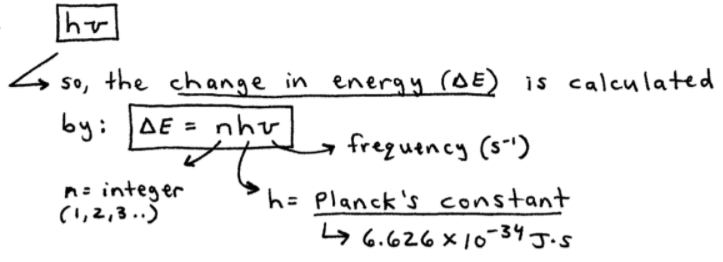As you can see in the above image,

h ➞ Planck's constant = 6.626 x 10-34 J.s
v ➞ the frequency = Hz, or s-1
n ➞ an integer = 1, 2, 3...

Because energy is transferred (absorbed or emitted) in these finite quantities, or "packets of energy," the energy is said to be quantized.

- quantized = only occurs in discrete units of size "hv"

-----

ex:  What quantum of energy is emitted by CuCl at a wavelength of 450. nm?

- use the given wavelength (λ) to find the frequency (v)
- then find the "packet" or "quantum" of energy using ΔE = nhv (with n = 1)...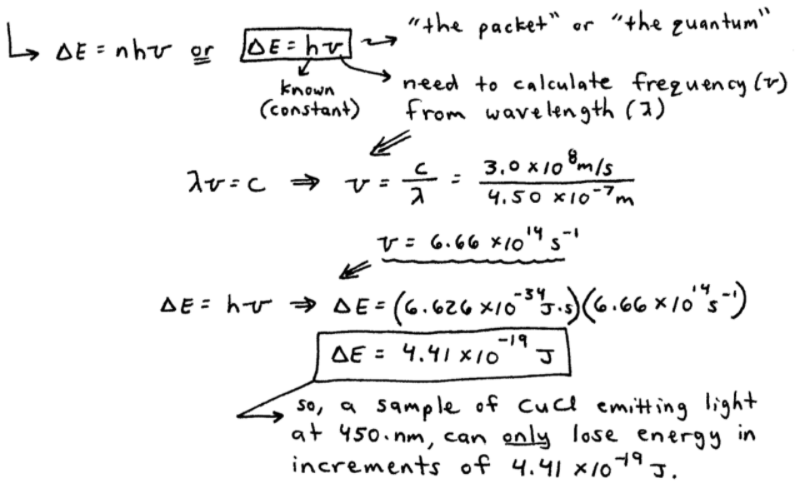=====

## Photons, and the Wave-Particle Duality of Light

Einstein continued with Planck's research (above), and suggested that EMR ("light") can exhibit the same type of behavior as exhibited by matter in Planck's above postulation.

Einstein proposed that EMR can be viewed as a stream of "particles" called photons.

- photons = a "packet" or "quantum" of light.

This proposal led to...

### The Wave-Particle Duality of Light

wave-particle duality of light = a phenomenon in which light can exhibit both wave-like and particle-like behavior.

➞ the energy of a photon is given by:  Ephoton = hv = hc / λ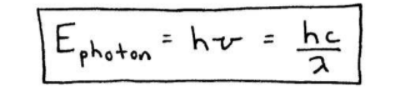=====

## The de Broglie Wavelength

After Einstein's wave-particle duality of light, scientists, including de Broglie, began considering if matter could also act as either a wave or a particle...

Matter, previously thought to behave (and travel through space) as a particle, can also show wave-like characteristics as well.

de Broglie = found a relationship between mass and wavelength:   λ = h/mv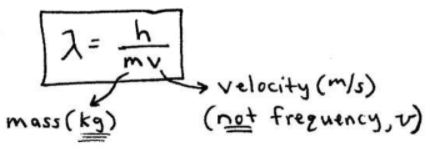Let's do an example...

ex:  Compare the wavelength for an electron (mass = 9.11 x 10-31 kg) traveling at a speed of 1.0 x 107 m/s with that for a ball (mass = 0.10 kg) traveling at 35 m/s. (1 J = 1 kg.m2 / s2).

-- we'll perform the calculations side by side, then compare...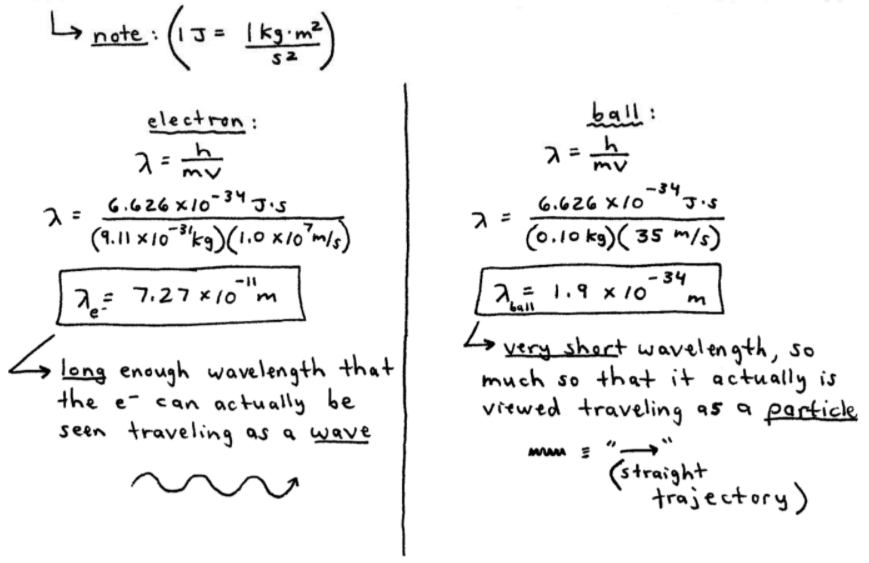=====

## Photoelectric Effect

Photoelectric Effect = the observation that many metals emit electrons when light shines upon them.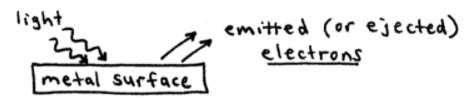### Threshold Frequency

The light used to dislodge the electrons must have a high enough energy such that it meets or exceeds the threshold frequency (Φ).

➞ a single photon must have enough energy (hv) to dislodge an electron which is bound to the metal with a binding energy = Φ

➞ so, the threshold frequency condition is:  hv  ≥  Φ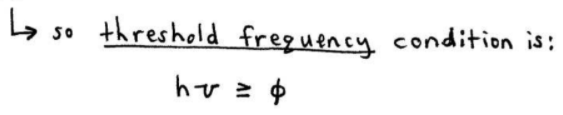=====

In my next post covering SECTION 7 - Quantum-Mechanical View of the Atom, and Periodicity,

We'll discuss the Atomic Spectrum of Hydrogen and the Bohr Model of the Atom.